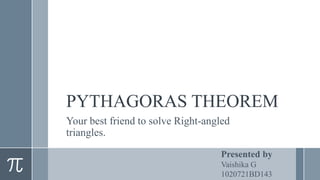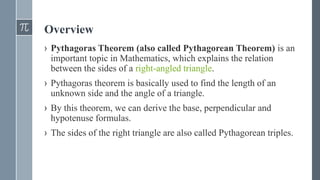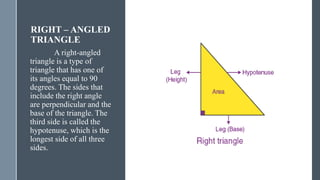Ce diaporama a bien été signalé.
Le téléchargement de votre SlideShare est en cours. ×

# PYTHAGORAS THEOREM.pptx

Publicité
Publicité
Publicité
Publicité
Publicité
Publicité
Publicité
Publicité
Publicité
Publicité
PublicitéChargement dans…3
×

## Consultez-les par la suite

1 sur 11 Publicité

# PYTHAGORAS THEOREM.pptx

Pythagoras Theorem (also called Pythagorean Theorem) is an important topic in Mathematics, which explains the relation between the sides of a right-angled triangle. The sides of the right triangle are also called Pythagorean triples. The formula and proof of this theorem are explained here with examples.

Pythagoras theorem is basically used to find the length of an unknown side and the angle of a triangle. By this theorem, we can derive the base, perpendicular and hypotenuse formulas.
Hypotenuse^2 = Perpendicular^2 + Base^2
c^2 = a^2 + b^2

Pythagoras Theorem (also called Pythagorean Theorem) is an important topic in Mathematics, which explains the relation between the sides of a right-angled triangle. The sides of the right triangle are also called Pythagorean triples. The formula and proof of this theorem are explained here with examples.

Pythagoras theorem is basically used to find the length of an unknown side and the angle of a triangle. By this theorem, we can derive the base, perpendicular and hypotenuse formulas.
Hypotenuse^2 = Perpendicular^2 + Base^2
c^2 = a^2 + b^2

Publicité
Publicité

### PYTHAGORAS THEOREM.pptx

1. 1. PYTHAGORAS THEOREM Your best friend to solve Right-angled triangles. Presented by Vaishika G 1020721BD143
2. 2. Overview › Pythagoras Theorem (also called Pythagorean Theorem) is an important topic in Mathematics, which explains the relation between the sides of a right-angled triangle. › Pythagoras theorem is basically used to find the length of an unknown side and the angle of a triangle. › By this theorem, we can derive the base, perpendicular and hypotenuse formulas. › The sides of the right triangle are also called Pythagorean triples.
3. 3. RIGHT – ANGLED TRIANGLE A right-angled triangle is a type of triangle that has one of its angles equal to 90 degrees. The sides that include the right angle are perpendicular and the base of the triangle. The third side is called the hypotenuse, which is the longest side of all three sides.
4. 4. Statement Pythagoras theorem states that “In a right-angled triangle, the square of the hypotenuse side is equal to the sum of squares of the other two sides“.
5. 5. Formula Consider the triangle given in the previous slide: Where “a” is the perpendicular, “b” is the base, “c” is the hypotenuse. According to the definition, the Pythagoras Theorem formula is given as: 2
6. 6. PROOF Given: A right-angled triangle ABC, right-angled at B. To Prove: AC2 = AB2 + BC2 Construction: Draw a perpendicular BD meeting AC at D.
7. 7. Proof › We know, △ADB ~ △ABC › Therefore, › 𝐴𝐷 𝐴𝐵 = 𝐴𝐵 𝐴𝐶 (corresponding sides of similar triangles) › Or, AB2 = AD × AC ……………………………..……..(1) › Also, △BDC ~△ABC › Therefore, › 𝐶𝐷 𝐵𝐶 = 𝐵𝐶 𝐴𝐶
8. 8. Proof › Or, BC2= CD × AC ……………………………………..(2) › Adding the equations (1) and (2) we get, › AB2 + BC2 = AD × AC + CD × AC › AB2 + BC2 = AC (AD + CD) › Since, AD + CD = AC › Therefore, AC2 = AB2 + BC2 › Hence, the Pythagorean theorem is proved.
9. 9. Application • To know if the triangle is a right-angled triangle or not. • In a right-angled triangle, we can calculate the length of any side if the other two sides are given. • To find the diagonal of a square.
10. 10. Wrapping it up  The Pythagorean Theorem can be used only on _____triangles.  When should the Pythagorean Theorem be used?  What should be done first when solving a word problem involving the Pythagorean Theorem?  What must be done before writing the answer to a Pythagorean Theorem problem?  Right  When the length of 2 sides are known and the length of 3rd side is needed  Draw and label triangle  Check to see whether the answer should be rounded or not